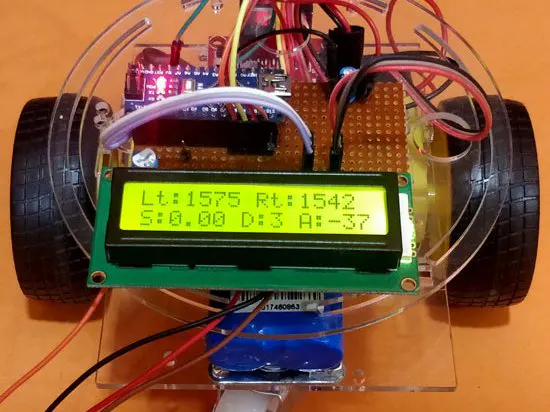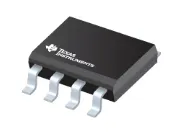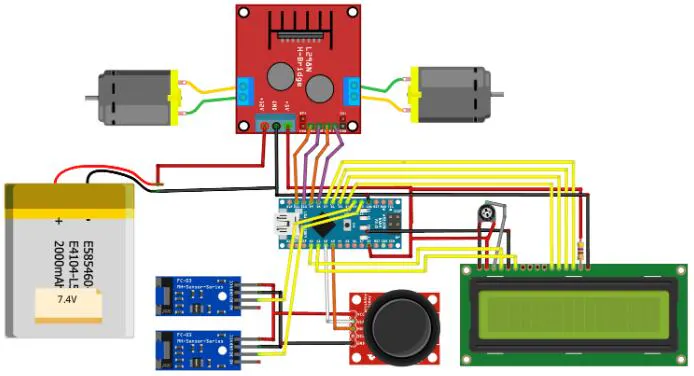Measurement of Mobile Robots with Arduino and LM393 Sensors

This project can help you measure the speed, distance and angle of the robot.Things used in this project

Hardware componentsTexas Instruments LM393 chip
×1

Software apps and online servicesArduino IoT Cloud

Hand tools and fabrication machinesSolder Pot, LCD Display

Schematics

schematic_pEct78xgP9.jpgCode

Untitled file

Arduino
#define LM_pos 9       // left motor
#define LM_neg 8       // left motor
#define RM_pos 10       // right motor
#define RM_neg 11       // right motor
#define joyX A2
#define joyY A3
void setup()
{
rotation = rpm = pevtime = 0; //Initialize all variable to zero
Serial.begin(9600);
lcd.begin(16, 2); //Initialise 16*2 LCD
lcd.print("Bot Monitor"); //Intro Message line 1
lcd.setCursor(0, 1);
lcd.print("-CircuitDigest "); //Intro Message line 2
delay(2000);
lcd.clear();
lcd.print("Lt:     Rt:    ");
lcd.setCursor(0, 1);
lcd.print("S:     D:  A:   ");

pinMode(LM_pos, OUTPUT);
pinMode(LM_neg, OUTPUT);

pinMode(RM_pos, OUTPUT);
pinMode(RM_neg, OUTPUT);

digitalWrite(LM_neg, LOW);
digitalWrite(RM_neg, LOW);

attachInterrupt(digitalPinToInterrupt(2), Left_ISR, CHANGE); //Left_ISR is called when left wheel sensor is triggered
attachInterrupt(digitalPinToInterrupt(3), Right_ISR, CHANGE);//Right_ISR is called when right wheel sensor is triggered
}
void Left_ISR()
{
left_intr++;delay(10);
}

void Right_ISR()
{
right_intr++; delay(10);

rotation++;
dtime=millis();
if(rotation>=40)
{
timetaken = millis()-pevtime; //timetaken in millisec
rpm=(1000/timetaken)*60;    //formulae to calculate rpm
pevtime = millis();
rotation=0;
}
}

int acceleration = map (xValue, 500, 0, 0, 200);

if (xValue<500)
{
analogWrite(LM_pos, acceleration);
analogWrite(RM_pos, acceleration);
}

else
{
analogWrite(LM_pos, 0);
analogWrite(RM_pos, 0);
}

if (yValue>550)
analogWrite(RM_pos, 80);

if (yValue<500)
analogWrite(LM_pos, 100);
v = radius_of_wheel * rpm * 0.104; //0.033 is the radius of the wheel in meter
int angle_left = (left_intr % 360) * (90/80) ;
int angle_right = (right_intr % 360) * (90/80) ;
angle = angle_right - angle_left;

lcd.setCursor(3, 0); lcd.print("    "); lcd.setCursor(3, 0); lcd.print(left_intr);
lcd.setCursor(11, 0); lcd.print("    "); lcd.setCursor(11, 0);lcd.print(right_intr);
lcd.setCursor(2, 1); lcd.print("  "); lcd.setCursor(2, 1);lcd.print(v);
lcd.setCursor(9, 1); lcd.print("  "); lcd.setCursor(9, 1);lcd.print(distance);
lcd.setCursor(13, 1); lcd.print("   "); lcd.setCursor(13, 1);lcd.print(angle);